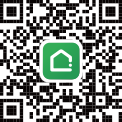• 净月区
• 德惠市
• 宽城区
• 二道区
• 绿园区
• 朝阳区
• 双阳区
• 榆树市
• 九台区
• 农安县
• 经开北区
• 经开区
• 高新区
• 汽车产业开发区
• 南关区
• 高新北区
• 公主岭市
• 世界雕塑公园
• 净月区
• 双阳区
• 净水厂
• 临河街
• 北海公园
• 东方广场南
• 二道区周边
• 德惠市
• 榆树市
• 农安县
• 合隆经济开发区
• 宽城区周边
• 九台
• 德惠市
• 客车厂
• 合隆经济开发区
• 人民广场西
• 大经路
• 东大桥
• 和顺
• 住邦城市广场
• 长新街
• 站前
• 小南
• 八里堡
• 天光路
• 小城子
• 北环沿线
• 柳影
• 四季青市场
• 工业大学北湖校区
• 宽城区周边
• 绿园区周边
• 北湖
• 净月区
• 双阳区
• 平阳
• 劳动公园
• 万通
• 欧美工业园
• 东方广场北
• 东大桥
• 和顺
• 亚泰
• 十里堡
• 二道区周边
• 小南
• 后十里堡
• 八里堡
• 天光路
• 宽城区周边
• 九台
• 北湖
• 红旗
• 农安县
• 普阳
• 客车厂
• 合隆经济开发区
• 人民广场西
• 支农东
• 支农西
• 住邦城市广场
• 站前
• 小城子
• 北环沿线
• 西部新城开发区
• 西安广场
• 天嘉
• 四联大街
• 四季青市场
• 景阳广场
• 宽城区周边
• 绿园区周边
• 范家屯
• 净月区
• 红旗
• 高新
• 友谊公园
• 桂林路
• 人民广场西
• 南湖
• 肿瘤医院
• 欧亚卖场
• 吉大前进校区
• 硅谷街北
• 硅谷街南
• 南部新城
• 吉大南岭校区
• 东风
• 长沈路
• 汽车广场
• 站前
• 景阳广场
• 富峰
• 朝阳区周边
• 净月区
• 双阳区
• 二道区周边
• 德惠市
• 榆树市
• 德惠市
• 榆树市
• 二道区周边
• 宽城区周边
• 九台
• 德惠市
• 农安县
• 合隆经济开发区
• 宽城区周边
• 绿园区周边
• 二道区周边
• 后十里堡
• 奋进
• 宽城区周边
• 九台
• 北湖
• 净月区
• 净水厂
• 临河街
• 吉大南岭校区
• 劳动公园
• 万通
• 欧美工业园
• 北海公园
• 东方广场南
• 东方广场北
• 十里堡
• 二道区周边
• 高新
• 友谊公园
• 南湖
• 肿瘤医院
• 欧亚卖场
• 吉大前进校区
• 硅谷街北
• 硅谷街南
• 南部新城
• 长沈路
• 富峰
• 范家屯
• 红旗
• 高新
• 欧亚卖场
• 硅谷街北
• 硅谷街南
• 东风
• 支农东
• 支农西
• 长沈路
• 汽车广场
• 四联大街
• 景阳广场
• 富峰
• 宽城区周边
• 西潘家屯
• 绿园区周边
• 世界雕塑公园
• 南四环
• 净月区
• 友谊公园
• 人民广场西
• 南湖
• 吉大前进校区
• 硅谷街北
• 硅谷街南
• 南部新城
• 净水厂
• 临河街
• 吉大南岭校区
• 平阳
• 劳动公园
• 大经路
• 东大桥
• 和顺
• 站前
• 天光路
• 朝阳区周边
• 德惠市
• 二道区周边
• 小南
• 小城子
• 工业大学北湖校区
• 奋进
• 宽城区周边
• 九台
• 北湖
• 1号线
• 2号线
• 3号线
• 4号线
• 8号线

• 5000元/m²以下
• 5000-6000元/m²
• 6000-7000元/m²
• 7000-8000元/m²
• 8000-10000元/m²
• 10000-15000元/m²
• 15000元/m²以上
• - 确定
+ 更多及自定义

• 50万以下
• 50-80万
• 80-100万
• 100-120万
• 120-150万
• 150-200万
• 200-300万
• 300万以上
• - 万元 确定
+ 更多及自定义

• 50㎡以下
• 50-70㎡
• 70-90㎡
• 90-110㎡
• 110-130㎡
• 130-150㎡
• 150-200㎡
• 200㎡以上
• - 确定
+ 更多及自定义

• 1居
• 2居
• 3居
• 4居
• 5居
• 5居+

• 近期开盘
• 未来一个月
• 未来三个月
• 未来半年
• 过去一个月
• 过去三个月

• 住宅
• 别墅
• 商业
• 写字楼
• 底商

• 在售
• 未开盘
• 售罄

• 带装修
• 毛坯

• 优惠楼盘
• 品牌房企
• 地铁沿线
• 公交直达
• 小户型
• 现房
• 低单价
• 开发区
• 线上购
• 无理由退房
• 视频看房

• 默认排序
• 均价
• 开盘时间

 我关注的房源 我关注的房源 下载掌上链家链家APP 反馈/投诉 反馈/投诉